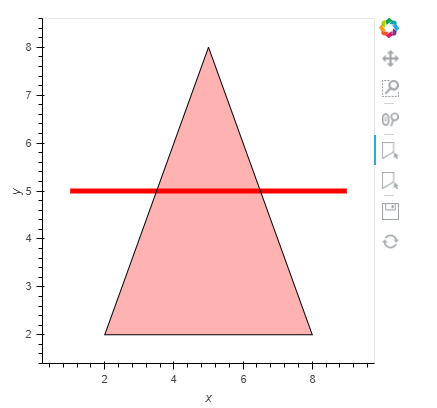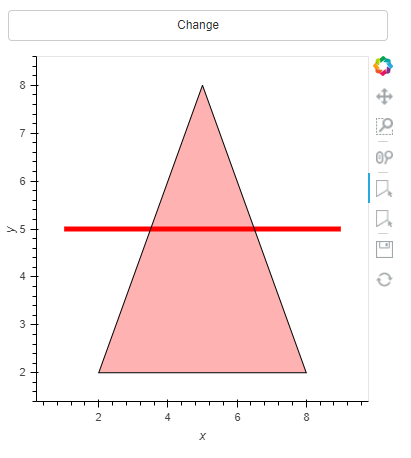# How do I programmatically change the underlying data in the PolyDraw stream

From the docs:

``````import holoviews as hv
from holoviews import streams, opts
path = hv.Path([[(1, 5), (9, 5)]])
poly = hv.Polygons([[(2, 2), (5, 8), (8, 2)]])
path_stream = streams.PolyDraw(source=path, drag=True, show_vertices=True)
poly_stream = streams.PolyDraw(source=poly, drag=True, num_objects=4,
show_vertices=True, styles={
'fill_color': ['red', 'green', 'blue']
})

(path * poly).opts(
opts.Path(color='red', height=400, line_width=5, width=400),
opts.Polygons(fill_alpha=0.3, active_tools=['poly_draw']))
``````

Now suppose I want to programmatically change the polygon to
`poly2 = hv.Polygons([[(1, 1), (4, 4), (8, 2)]])`

How would I do that?

If you do this:
`poly_stream.source = poly2`
it disconnects the original poly from the stream, so if you try to visualize `poly` like you did originally, you don’t get the drawing tools. You’d have to view `poly2` which would cause me to do a complete image reload.

This of course is terribly inefficient. Is there a better way that would allow me to modify the drawing without reload?

Ahh. I need to add `hv.extension('bokeh')`.

``````import holoviews as hv
from holoviews import streams, opts
import panel as pn
hv.extension('bokeh')
path = hv.Path([[(1, 5), (9, 5)]])
poly = hv.Polygons([[(2, 2), (5, 8), (8, 2)]])
path_stream = streams.PolyDraw(source=path, drag=True, show_vertices=True)
poly_stream = streams.PolyDraw(source=poly, drag=True, num_objects=4,
show_vertices=True, styles={
'fill_color': ['red', 'green', 'blue']
})

plot = (path * poly).opts(
opts.Polygons(fill_alpha=0.3, active_tools=['poly_draw']),
opts.Path(color='red', height=400, line_width=5, width=400),
)

pn.Column(plot).servable()
``````I would have expected something like the below to work. But nothing happens when I click the button.

``````import holoviews as hv
from holoviews import streams, opts
import panel as pn
hv.extension('bokeh')
path = hv.Path([[(1, 5), (9, 5)]])
poly = hv.Polygons([[(2, 2), (5, 8), (8, 2)]])
path_stream = streams.PolyDraw(source=path, drag=True, show_vertices=True)
poly_stream = streams.PolyDraw(source=poly, drag=True, num_objects=4,
show_vertices=True, styles={
'fill_color': ['red', 'green', 'blue']
})

plot = (path * poly).opts(
opts.Polygons(fill_alpha=0.3, active_tools=['poly_draw']),
opts.Path(color='red', height=400, line_width=5, width=400),
)
def update(*_):
print(poly.data)
poly.data = [[(1, 1), (4, 4), (8, 2)]]
print(poly.data)

button = pn.widgets.Button(name="Change")
button.on_click(callback=update)

pn.Column(button, plot).servable()
``````I’ve also tried to disable the streams but still clicking the button does change anything.

Something like the below reset the `poly`. But I don’t know if you want to keep anything the user has drawn?

``````from os import linesep
import holoviews as hv
from holoviews import streams, opts
import panel as pn
from random import randint

LINE = "red"
FILL1 = "green"
FILL2 = "fulvous"
FILL3 = "blue"

hv.extension('bokeh')
path = hv.Path([[(1, 5), (9, 5)]])
poly = hv.Polygons([[(2, 2), (5, 8), (8, 2)]])
path_stream = streams.PolyDraw(source=path, drag=True, show_vertices=True)
poly_stream = streams.PolyDraw(source=poly, drag=True, num_objects=4,
show_vertices=True, styles={
'fill_color': [FILL1, FILL2, FILL3]
})

def get_plot(path, poly):
return (path * poly).opts(
opts.Polygons(fill_alpha=0.3, active_tools=['poly_draw']),
opts.Path(color=LINE, height=400, line_width=5, width=400),
)
plot = get_plot(path,poly)
plot_pane = pn.pane.HoloViews(plot)

def update(*_):
poly2 = hv.Polygons([[(randint(2,5), randint(6,9)), (4, 4), (8, 2)]])
poly_stream.source = poly2
plot_pane.object = get_plot(path, poly2)

button = pn.widgets.Button(name="RANDOM RESET")
button.on_click(callback=update)

pn.Column(plot_pane,  button).servable()
``````